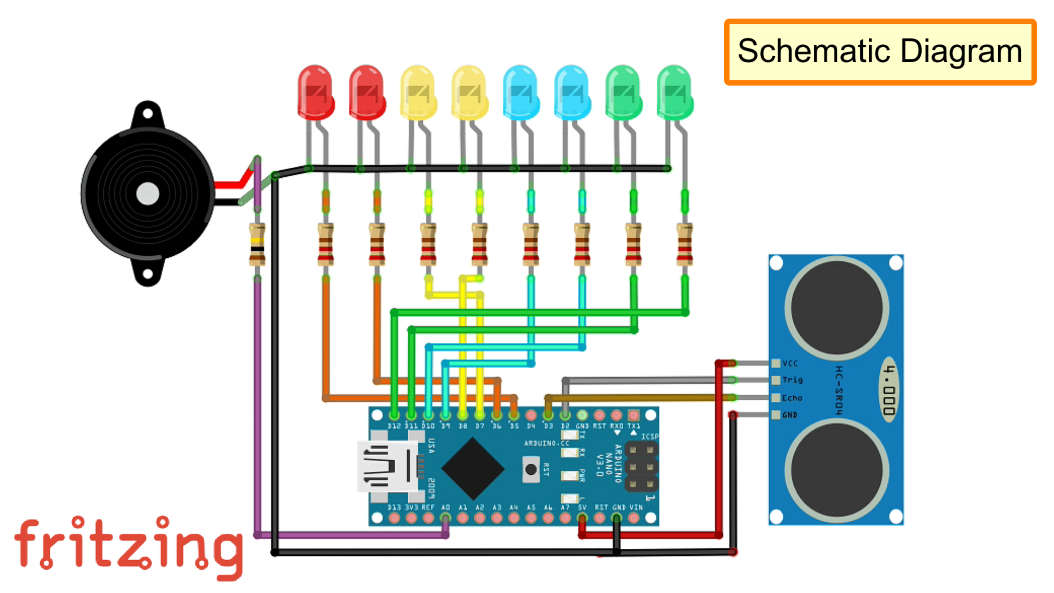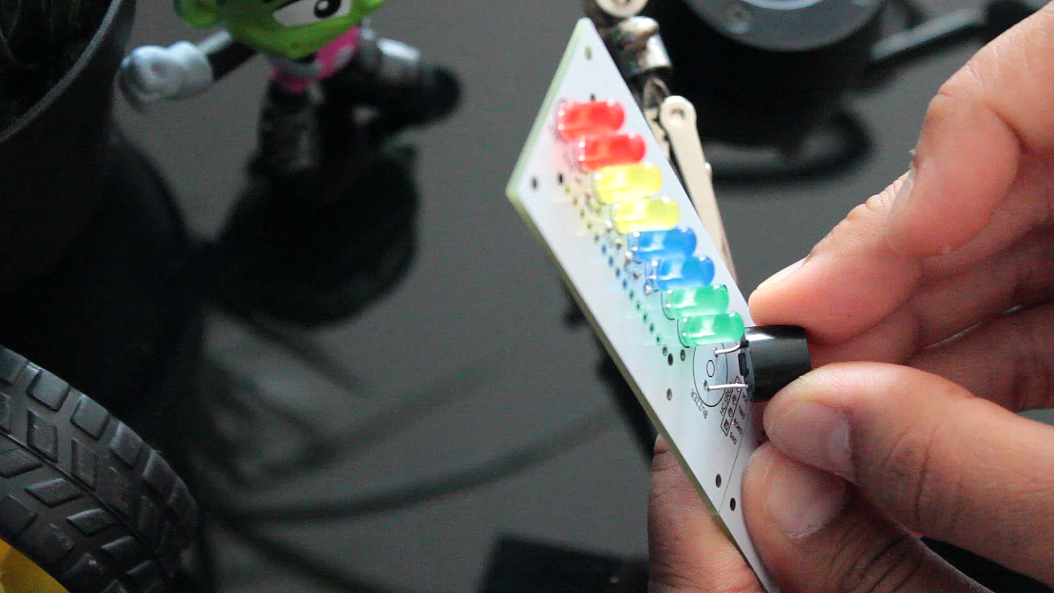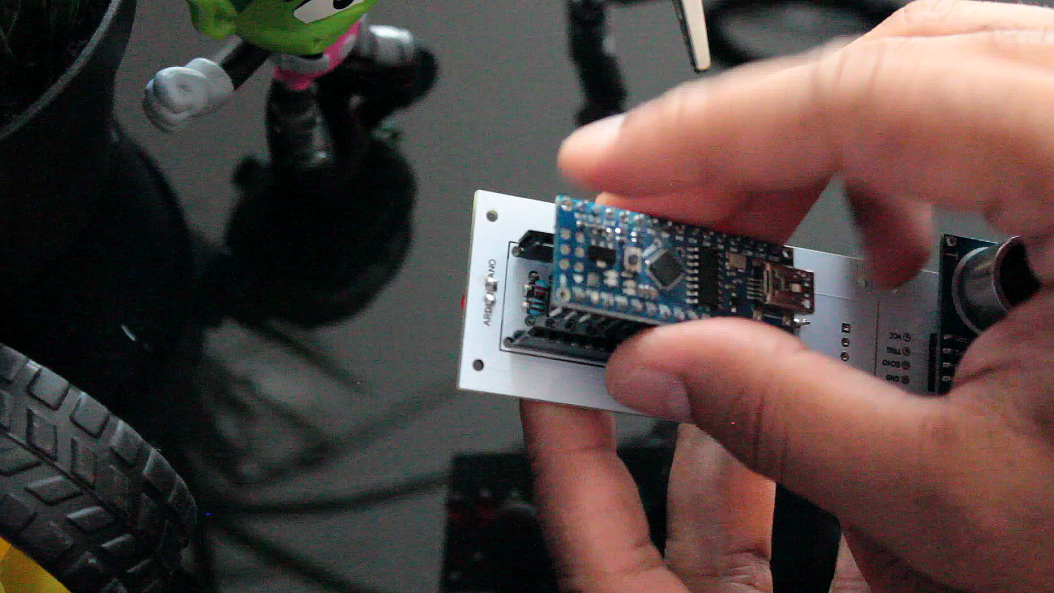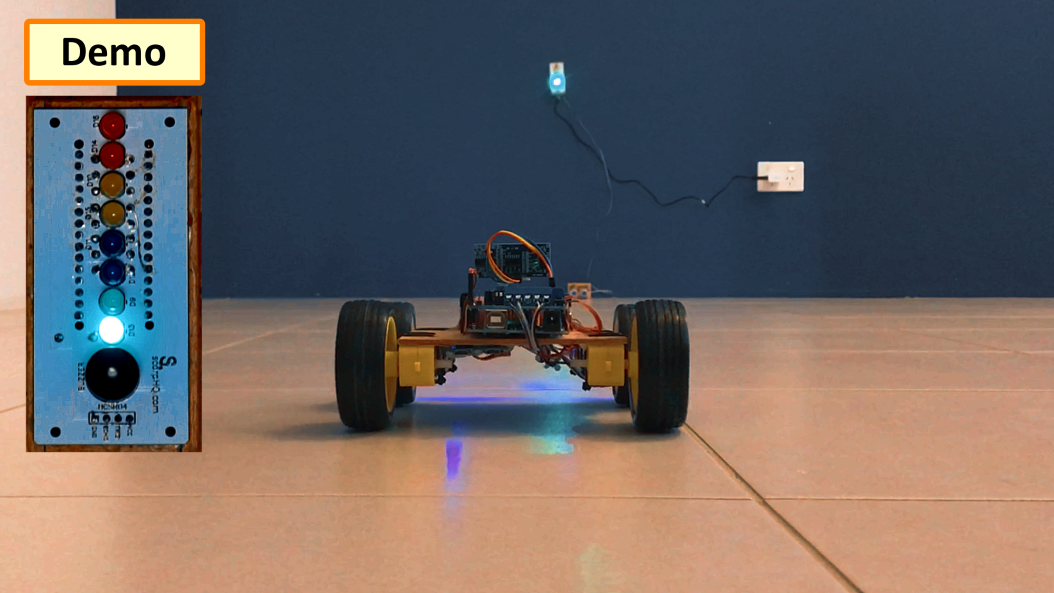Arduino

# DIY - Arduino Based Parking Assistant V2December 07, 2019 by Ashish Adhikari
ShareIn this tutorial, I am going to use an ultrasonic sensor to calculate the car's distance from the garage wall

When life gives you bananas!!!!! Just eat them.

Necessity is the mother of inventions, and I won't deny that fact. Frankly speaking, this is the 2nd time I bumped into our garage wall since we moved into this new house. That's it, there would be no third time.

In this video, I am going to use an ultrasonic sensor to calculate the car's distance from the garage wall and display it using green, blue, yellow and red LEDs. The color of LEDs indicates whether to keep moving, slow down, stop or go back.

The total cost of the project is around \$20 - \$25.

## SchematicFor this project we need:

• 8 x Multi Colored LEDs
• 8 x 220ohm Resistors
• 1 x Arduino NANO
• 1 x HC-SR04 Ultrasonic Sensor
• 1 x Speaker and
• 1 x 100ohm Resistor

Lets start by connecting the LEDs to the Pin number D5 to D12 of the Arduino with a 200ohm resistor in between each of the pins. Then, lets connect the speaker to A0 pin of the Arduino. The TRIG pin of the Ultrasonic Sensor connects to D2 and the ECHO pin connects to the D3 pins of the Arduino. Finally, connect the VCC pin of the Ultrasonic Sensor to the 5V output of the Arduino and to finish the circuit connect all the -ve pins to the GND pin of the Arduino.

## Components AssemblyI will start by soldering the LEDs to the board. Red on top, then yellow followed by blue and green at the bottom.

You can use whatever color you want, totally depend on how you want to present it. The reason I chose these colors was to show the level of severity as the car approaches the wall. I could have even used a single color for the whole setup.

After soldering the LEDs I am soldering the 8 x 220ohm current limiting resistors to the back of the board.

Next, I am soldering the buzzer and the 100ohm resistor to board.

After that I am soldering 2 rows of Female Pin Header Strips to hold the Arduino.

Next, its time for me to solder the ultrasonic sensor to the bottom bit of the board.

Finally before de-attaching the bottom bit I am soldering the cables to the board.

Alright, so this is how it looks like. Now, lets look at the code in the next section.

## The CodeStart the code by including the "NewTone.h" library and by defining the constants and the global variables that will be used throughout the code.

Then in the setup section define the pin modes. Now, in the loop section calculate the "Distance" in inches by reading the value received from the Ultrasonic Sensor. Then by checking the value of the "Distance" we will turn on or off the LEDs based on how far the object is. If the distance is greater than 200 turn all the LEDs and the buzzer off as the object is out of range.

Next bit of the code checks if the object is currently stationary. It compares the value of current distance with the previous distance and if the values are same (object hasn't moved) it increments a counter. If the object moves any-time during this process the counter is reset to 0.

When the counter reaches 20 all the LEDs are turned off. And finally create the function that turns off all the LEDs and the buzzer.

## DemoUsing my Land Rover R1V2 I am going to demonstrate the project to you guys. As you can see the LED indicators go from green to red as the rover approaches the ultrasonic sensor. Yes!! mission accomplished.

## Thanks

Thanks again for checking my post. I hope it helps you.

If you want to support me subscribe to my YouTube Channel (https://www.youtube.com/user/tarantula3).

Thanks, ca again in my next tutorial.

JLCPCB - 2\$ For PCB Prototype: https://jlcpcb.com

• V1: https://diyfactory007.blogspot.com/2018/04/diy-arduino-based-car-parking-assistant.html
• V2: https://diyfactory007.blogspot.com/2019/12/diy-arduino-based-parking-assistant-v2.html
• Teaser: https://youtu.be/UoEEZBV6NOg
• Video V1: https://youtu.be/LQGhprwuHe0
• Video V2: https://youtu.be/MqJxUf3Cugg
``````#include <NewTone.h>

int trigPin = 2; // Sensor Trip pin connected to Arduino pin D5
int echoPin = 3; // Sensor Echo pin connected to Arduino pin D6
int r1LED  = 5;
int r2LED  = 6;
int y1LED  = 7;
int y2LED  = 8;
int b1LED  = 9;
int b2LED  = 10;
int g1LED  = 11;
int g2LED  = 12;
int buzzer = A0; // Buzzer connected to Analogue pin A0
long TempDistance = 0; // A variable to store the temporary distance
int counter = 0; // Counter value to check if the object has stopped moving

void setup() {
Serial.begin(115200);
pinMode(trigPin, OUTPUT);
pinMode(echoPin, INPUT);
pinMode(r1LED,   OUTPUT);
pinMode(r2LED,   OUTPUT);
pinMode(y1LED,   OUTPUT);
pinMode(y2LED,   OUTPUT);
pinMode(b1LED,   OUTPUT);
pinMode(b2LED,   OUTPUT);
pinMode(g1LED,   OUTPUT);
pinMode(g2LED,   OUTPUT);
pinMode(buzzer,  OUTPUT);
}

void loop() {
long duration, Distance;
digitalWrite(trigPin, LOW);   delayMicroseconds(2);
digitalWrite(trigPin, HIGH);  delayMicroseconds(10);
digitalWrite(trigPin, LOW);
duration = pulseIn(echoPin, HIGH);
Distance = (duration/2) / 74; // Distance in Inches

if(counter < 20){ // Do the rest if the car is still moving
if (Distance > 200) { turnThemAllOff(); } // Nothing in the garrage
if (Distance <= 110){ digitalWrite(g2LED, HIGH); } else { digitalWrite(g2LED, LOW); } // Turn on the 1st Green LED
if (Distance <= 80) { digitalWrite(g1LED, HIGH); } else { digitalWrite(g1LED, LOW); } // Turn on the 2nd Green LED
if (Distance <= 70) { digitalWrite(b2LED, HIGH); } else { digitalWrite(b2LED, LOW); } // Turn on the 1st Blue LED
if (Distance <= 60) { digitalWrite(b1LED, HIGH); } else { digitalWrite(b1LED, LOW); } // Turn on the 2nd Blue LED
if (Distance <= 50) { digitalWrite(y2LED, HIGH); } else { digitalWrite(y2LED, LOW); } // Turn on the 1st Yellow LED
if (Distance <= 40) { digitalWrite(y1LED, HIGH); } else { digitalWrite(y1LED, LOW); } // Turn on the 2nd Yellow LED
if (Distance <= 30) { digitalWrite(r2LED, HIGH); } else { digitalWrite(r2LED, LOW); } // Turn on the 1st Red LED
if (Distance <= 20) { digitalWrite(r1LED, HIGH); } else { digitalWrite(r1LED, LOW); } // Turn on the 2nd Red LED
if (Distance <  10) { NewTone(buzzer, 500); }      else { noNewTone(buzzer); }        // Item is way to close - Start the buzzer
delay(500);
};

if ((Distance == TempDistance) || ((Distance+1) == TempDistance) || ((Distance-1) == TempDistance)){
if(counter >= 20){ // Turn off the lights if the object hasn't moved for 20 cycles (no change in distance)
Serial.println("No movement detected, turning off the lights");
turnThemAllOff();
} else { counter++;   };
} else   { counter = 0; }; // Reset counter if there is a movement

TempDistance = Distance;
Serial.print(Distance);     Serial.println(" inches");
Serial.print("Counter : "); Serial.println(counter);
}

// Function to turn the LEDs off
void turnThemAllOff(){
digitalWrite(r1LED, LOW);
digitalWrite(r2LED, LOW);
digitalWrite(y1LED, LOW);
digitalWrite(y2LED, LOW);
digitalWrite(b1LED, LOW);
digitalWrite(b2LED, LOW);
digitalWrite(g1LED, LOW);
digitalWrite(g2LED, LOW);
noNewTone(buzzer);
}``````